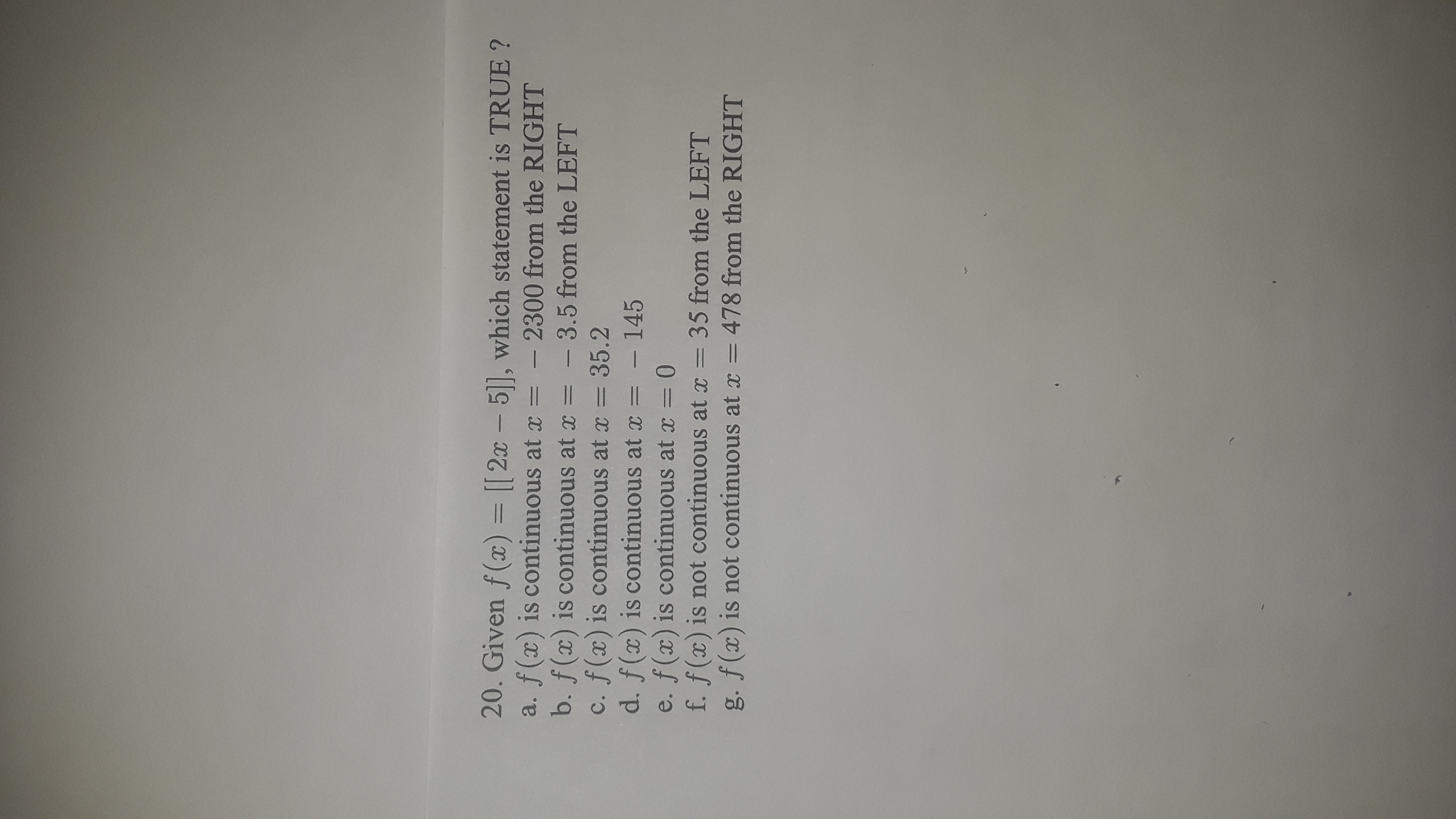# 20. Given f(x) = [[ 2x – 5|], which statement is TRUE ?a. f(x) is continuous at x =b. f(x) is continuous at x =c. f(x) is continuous at x = 35.2d. f(x) is continuous at x = – 145e. f(x) is continuous at = 0f. f(x) is not continuous at x = 35 from the LEFTg. f(x) is not continuous at x = 478 from the RIGHT%3D2300 from the RIGHT- 3.5 from the LEFT

Question
36 viewshelp_outlineImage Transcriptionclose20. Given f(x) = [[ 2x – 5|], which statement is TRUE ? a. f(x) is continuous at x = b. f(x) is continuous at x = c. f(x) is continuous at x = 35.2 d. f(x) is continuous at x = – 145 e. f(x) is continuous at = 0 f. f(x) is not continuous at x = 35 from the LEFT g. f(x) is not continuous at x = 478 from the RIGHT %3D 2300 from the RIGHT - 3.5 from the LEFT fullscreen
check_circle

Step 1

Double brackets or [[]] in math refer to rounding off the value inside to its greatest integer less than or equal to the value.

To check the limit from the Right of -2300 used limit x-> -2300^+

Step 2

On the right side of -4605 lets take -4604.99

Greatest integer less than or equal to -4604.99 is -4605.

Step 3

f(2300)=-4605 too.

So f(x) is continuous at x=-2300 fr...

### Want to see the full answer?

See Solution

#### Want to see this answer and more?

Solutions are written by subject experts who are available 24/7. Questions are typically answered within 1 hour.*

See Solution
*Response times may vary by subject and question.
Tagged in

### Other